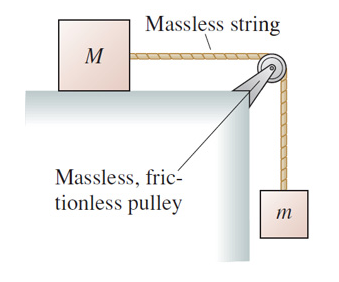# Problem: The block of mass M in the following figure slides on a frictionless surface. (Figure 1)Find an expression for the tension in the string.Express your answer in terms of the variables m, M, and appropriate constants.

###### FREE Expert Solution

Newton's second law:

$\overline{){\mathbf{\Sigma }}{\mathbf{F}}{\mathbf{=}}{\mathbf{m}}{\mathbf{a}}}$

We'll apply Newton's second law to each block. The tension in the rope is the same. The blocks have the same acceleration.

90% (331 ratings)###### Problem Details

The block of mass M in the following figure slides on a frictionless surface. (Figure 1)Find an expression for the tension in the string.

Express your answer in terms of the variables m, M, and appropriate constants.